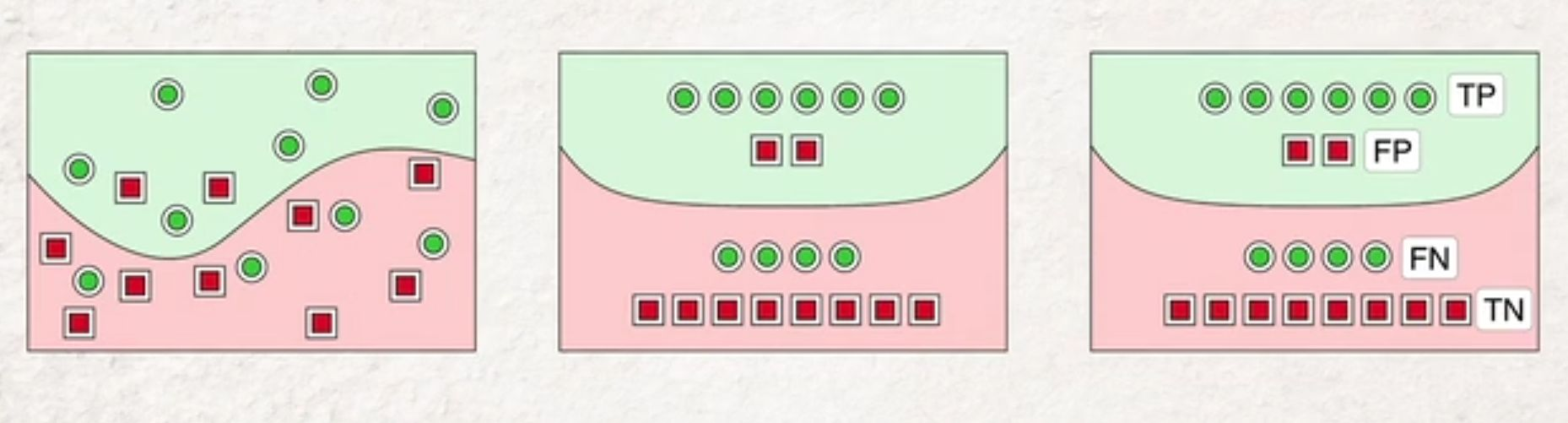Math and science::INF ML AI

# Accuracy, precision, recall[\begin{aligned} \text{accuracy} &= \frac{?}{?} \\ \text{precision} &= \frac{?}{?} \\ \text{recall} &= \frac{?}{?} \\ \end{aligned} ]

### Formulated in words

accuracy = [$$\frac{ \text{something} }{ \text{something} }$$ ]

precision = [...]

recall = [...]

### Pathalogical and benign examples

Are false positives dangerous? Are false negatives dangerous? Poor precision affords many false positives. Poor recall affords many false negatives. If the true/false meaning of any test is reversed, false positives become false negatives and vice versa.

• Poor precision is pathalogical: COVID19 testing where positive represents [having/being free of the virus?].
• Poor precision is benign: COVID19 testing, where a positive represents [having/being free of the virus?].
• Poor recall is pathalogical: COVID19 testing, where a positive represents [having/being free of the virus?].
• Poor recall is benign: COVID19 testing where positive represents [having/being free of the virus?].